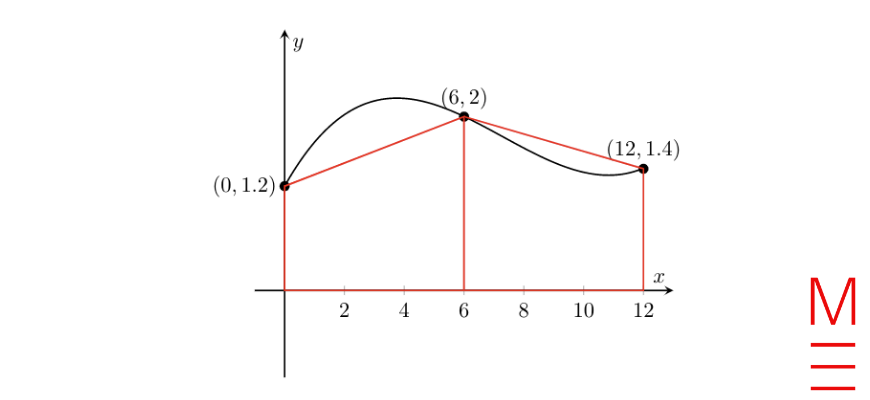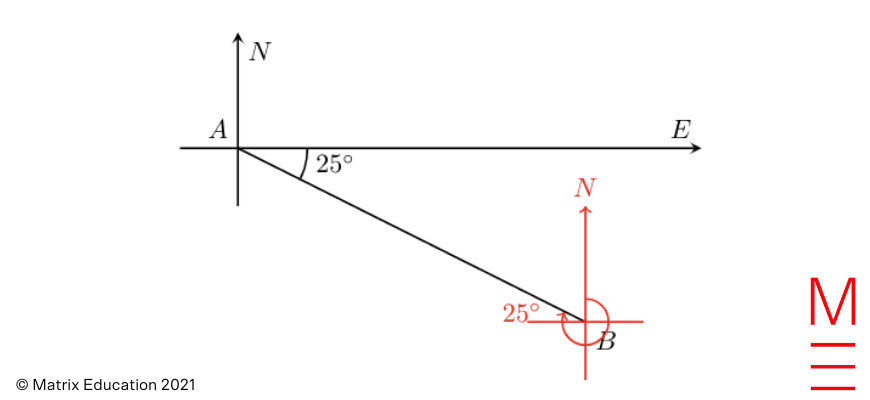# 2021 HSC Maths Standard 2 Exam Paper Solutions

The 2021 HSC Maths Std 2 Exam was on Monday 15 November. Read on to see how our Maths gurus would have answered things.The Matrix 2021 HSC Maths Standard 2 Exam Paper Solutions are here!

## 2021 HSC Maths Standard 2 Exam Paper Solutions

Have you seen the 2021 HSC Mathematics Standard 2 exam Paper yet?

In this post, we will work our way through the 2021 HSC Maths Standard 2 exam paper and give you the solutions, written by our Head of Mathematics Oak Ukrit and his team.

## Multiple-choice section

 Question Answer Explanation 1 A For option A, the perimeter is $$4 \times 8 = 32$$ cm. For option B, the perimeter is $$2 \left(3 + 11\right) = 28$$ cm. For option C, the perimeter is $$3 \times 10 = 30$$ cm. For option D, the perimeter is $$3 + 4 + 4 + 9 = 20$$ cm. 2 D Vertex A has degree 4, vertex B is degree 3, vertex C has degree 4, vertex D has degree 2, and vertex E has degree 3. So the sum of the degrees of all the vertices is $$4 + 3 + 4 + 2 + 3 = 16$$. 3 C $$25$$ appears twice, all the others appear once only, so the mode is $$25$$. 4 D Its salvage value is \begin{align*} S &= V_0 \left(1 – r\right)^n \\ &= \$2467 \left(1 – 0.15\right)^3 \\ &= \$1515 \end{align*} 5 B His hourly wage after four years will be \begin{equation*} \$21.50 \left(1 + \frac{0.021}{1}\right)^{1 \times 4} = \$21.50 \times \left(1.021\right)^4 \end{equation*} 6 A \begin{align*} a &= \frac{22}{7} \\ &= 3.14285 \dots \\ &= 3.14 \end{align*} 7 C Options A and D show that there are close to 100 downloads on day 1, which is not consistent with the graph given, so they are wrong. The graph given shows that the number of downloads increases from day 1 to day 10, and it decreases after that. Option B shows that the number of downloads every day is roughly the same, and that is inconsistent with the graph given. The graph in option C is consistent with all the features shown in the graph given. 8 D \begin{align*} z &= \frac{x – \mu}{\sigma} \\ 2 &= \frac{x – 63}{8} \\ 16 &= x – 63 \\ x &= 79 \end{align*} 9 C After subtracting $$8$$ from the number, we get $$x – 8$$. After multiply the result by $$3$$, we get $$3 \left(x – 8\right)$$.$$2$$ more than $$x$$ is $$x + 2$$. Since these two results are the same, we obtain \begin{equation*} 3 \left(x – 8\right) = x + 2 \end{equation*} 10 A When $$x = 0$$,\begin{align*} y &= 10 \left(0.8\right)^0 \\ &= 10 \left(1\right) \\ &= 10 \end{align*} so options B and D are wrong.Since $$0.8 < 1$$, $$y = 10 \left(0.8\right)^x$$ decreases as $$x$$ increases, which means that option A is correct. 11 D The probability that Kim and Sam choose chocolates with different centres is \begin{align*} P(\text{one P and one C}) &= \frac{3}{8} \times \frac{5}{7} + \frac{5}{8} \times \frac{3}{7} \\ &= \frac{15}{28} \end{align*} 12 B\begin{align*} \text{area} &\approx \frac{6}{2} \left(1.2 + 2\right) + \frac{6}{2} \left(2 + 1.4\right) \\ &= 19.8 \; \text{km}^2 \end{align*} 13 B Let $$C$$ be the number of cleaners, $t$ be the amount of time (in hours) it takes to clean the warehouse, and [/altex]k[/latex] be a constant. Then \begin{align*} C &= \frac{k}{t} \\ 8 &= \frac{k}{60} \\ k &= 480 \end{align*} \begin{equation*} ∴ C = \frac{480}{t} \end{equation*} When $$C = 10$$, \begin{align*} 10 &= \frac{480}{t} \\ t &= \frac{480}{10} \\ t &= 48 \end{align*} 14 DSince $$270^\circ + 25^\circ = 295^\circ$$, the true bearing of $$A$$ from $$B$$ is $$295^\circ$$. 15 B \begin{align*}\text{number of professional runners} &= 11400 \times \frac{3}{3 + 16} \\ &= 1800 \end{align*} and \begin{align*} \text{total number of amateurs} &= 11400 \times \frac{16}{3 + 16} \\ &= 9600 \end{align*}Also, \begin{align*} \text{number of amateurs who completed the race} &= 9600 – 600 \\ &= 9000 \end{align*}So, \begin{align*} \text{no.\ of professional runners who completed the race} : \text{no.\ of amateurs who completed the race} &= 1800 : 9000 \\ &= 1 : 5 \end{align*}

## Long Response Section

### Question 16

 \begin{align*} V &= \frac{1}{2} \times \left[\frac{4}{3} \pi \left(2\right)^3\right] \\ &= 16.75516082 \\ &= 16.8 \; \text{cm}^3 \quad \text{(1 d.p.)} \end{align*}

### Question 17

 \begin{align*} \text{IQR} &= Q_3 – Q_1 \\ &= 10 – 4 \\ &= 6 \end{align*}and\begin{align*} Q_3 + 1.5 \times IQR &= 10 + 1.5 \times 6 \\ &= 19 \end{align*}Since $$20 > 19$$, it is an outlier.

### Question 20

We are only concerned about the longitude, i.e. how far East or West. Since $$151^\circ – 16^\circ = 135^\circ$$,

 \begin{align*} \text{time difference} &= \frac{135^\circ}{15} \\ &= 9 \, \text{hours (Australia is earlier)} \end{align*}So Robert should start at 2AM on Friday.

### Question 21

\begin{align*}
\text{interest rate} &= \frac{18.75}{12500} \\
&= 0.0015 \\
&= 0.15 \%
\end{align*}In month 7,\begin{align*}
\text{monthly interest} &= \$15624.20 \times 0.0015 \\ &= \$23.44
\end{align*}and\begin{align*}
\text{amount at the end of month 7} &= \$15624.2 + \$23.44 + \$500 \\ &= \$16147.64
\end{align*}So the final row of the table is as follows:

 Month Amount in account at beginning of month Monthly interest Deposit end of month 7 15624.20 23.44 500 16147.64

### Question 26b

 \begin{align*} \text{FV} &= \text{PV} + \text{I} \quad \text{(simple interest)} \\ \text{FV} &= \text{PV} + Prt \\ 37158.72 &= 35000 + 35000 \times r \times 1 \\ 2158.72 &= 35000r \\ r &= \frac{2158.72}{35000} \\ r &= 0.06167 \dots \\ r &= 6.17 \%  \text{( 2 d.p.)} \end{align*}

### Question 27a

 For Television B, \begin{align*} \text{hourly cost of electricity} &= 160 \, \text{W} \times \frac{25 \, \text{c}}{1000 \, \text{W}} \\ &= 4 \, \text{c} \end{align*} and \begin{align*} \text{annual cost of electricity} &= 4 \times 3 \times 365 \\ &= 4380 \, \text{c} \\ &= \43.80 \end{align*} so \begin{align*} \text{difference in the annual cost of electricity} &= \48.18 – \$43.80 \\ &= \$4.38 \quad \text{(Television B is cheaper)} \end{align*}

### Question 27b

 Let $$n$$ be the number of years it will take. \begin{align*} 900 + 48.18n &= 921.9 + 43.8n \\ 4.38n &= 21.9 \\ n &= 5 \end{align*} So it will take 5 years.

### Question 28a

Set calculator to STAT mode, select “A + Bx” and enter data points into table.

The $$y$$-intercept is $$2$$ and the gradient is $$3.2$$.

Hence, the equation of the least-squares regression line is $$y = 3.2 x + 2$$;

### Question 28b

The data point (10,45) would be above the regression line in the diagram, so the gradient would increase.

Question 29

 \begin{align*} x + \frac{x – 1}{2} &= 9 \\ \frac{2x + x – 1}{2} &= 9 \\ 3x – 1 &= 18 \\ 3x &= 19 \\ x &= \frac{19}{3} \end{align*}

### Question 30

 \begin{align*} \text{dividend yield} &= \frac{810}{1500 \times 27} \\ &= 0.02 \\ &= 2\% \end{align*}

### Question 41

Let $$X$$ be a female’s height in the population and $$Y$$ be a male’s height.

\begin{align*}
P(X< 175) &= 97.5\% \\
P(X < 160.6) &= 16\% \\
P(Y < h) &= 84\%
\end{align*}

Our aim is the find $$h$$. Use the empirical rule with the information given above.

\begin{align*}
\mu + 2\sigma &= 175 & \text{(1)} \\
\mu – \sigma &= 160.6 & \text{(2)}
\end{align*}

Subtracting equation (1) by (2) gives

\begin{align*}
3 \sigma &= 14.4 \\
∴ \sigma &= 4.8
\end{align*}

Substitute $$\sigma = 4.8$$ into (1),

\begin{align*}
\mu + 2(4.8) &= 175 \\
\mu = 165.4
\end{align*}

Hence, for the adult male population, the mean is $$1.05 \mu = 173.67$$ and the standard deviation is $$1.1 \sigma = 5.28$$.

Since being 84 % taller than the male population is 1 standard deviation above the mean, then we can calculate $$h$$ as

\begin{align*}
h &= 1.05 \mu + 1.1 \sigma \\
&= 173.67 + 5.28 \\
&= 178.95 \; \text{cm}
\end{align*}